# Algebra II : Adding and Subtracting Logarithms

## Example Questions

1 2 3 5 Next →

### Example Question #41 : Adding And Subtracting Logarithms

Combine the logs as one: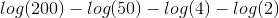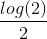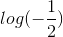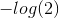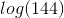Explanation:

Evaluate each term.  Write the property of logs when they are subtracted.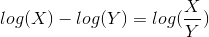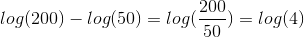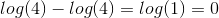The remaining term is:The answer is:### Example Question #42 : Adding And Subtracting Logarithms

Solve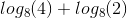.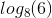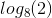Explanation:

When you add logarithms of the same base, you multiply the terms inside the log: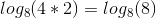A logarithm with a base that's the same as the term inside it is always equal to.

### Example Question #43 : Adding And Subtracting Logarithms

Solve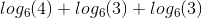.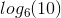Explanation:

When adding logs with the same base, we multiply the terms inside of them: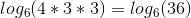Now we can expand the log again so that the terms inside of it match the base: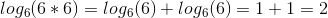### Example Question #44 : Adding And Subtracting Logarithms

Combine as one log: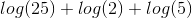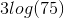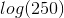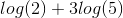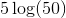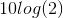Explanation:

According to log rules, whenever we are adding the terms of the logs, we can simply combine the terms as one log by multiplication.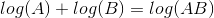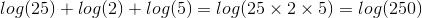The answer is:### Example Question #45 : Adding And Subtracting Logarithms

Simplify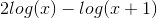.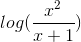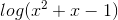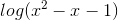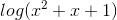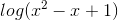Explanation:

First we can make the coefficient from the left term into an exponent: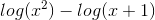Next, remember that if we're subtracting logs, we divide the terms inside them:### Example Question #46 : Adding And Subtracting Logarithms

Simplify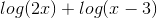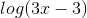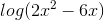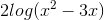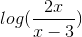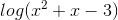Explanation:

When we add logs, we multiply the terms in them: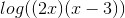From here, we multiply them out:### Example Question #47 : Adding And Subtracting Logarithms

True or false: for all positive values of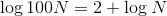False

True

True

Explanation:

By the Product of Logarithms Property,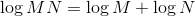Setting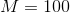, this becomes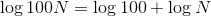"log" refers to the common, or base ten, logarithm, so, by definition,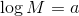if and only if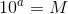.

Setting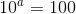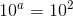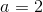,

so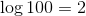and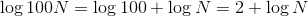.

The statement is true.

### Example Question #48 : Adding And Subtracting Logarithms

True or false: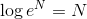for all values of.

True

False

False

Explanation:

A statement can be proved to not be true in general if one counterexample can be found. One such counterexample assumes that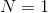. The statementbecomes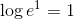or, equivalently,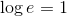The word "log" indicates a common, or base ten, logarithm, as opposed to a natural, or base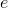, logarithm. By definition, the above statement is true if and only if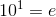or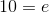.

This is false, sodoes not hold for. Since the statement fails for one value, it fails in general.

### Example Question #49 : Adding And Subtracting Logarithms

True or false: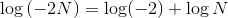for all negative values of.

False

True

False

Explanation:

It is true that by the Product of Logarithms Property,.

However, this only holds true if bothandare positive. The logarithm of a negative number is undefined, so the expression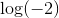is undefined. The statement is therefore false.

### Example Question #50 : Adding And Subtracting Logarithms

True or false: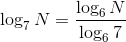for all positive.

False

True

True

Explanation:

By the Change of Base Property of Logarithms, if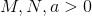and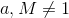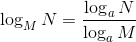Substituting 7 forand 6 for, the statement becomes the given statement.

The correct choice is "true."

1 2 3 5 Next →

### All Algebra II Resources